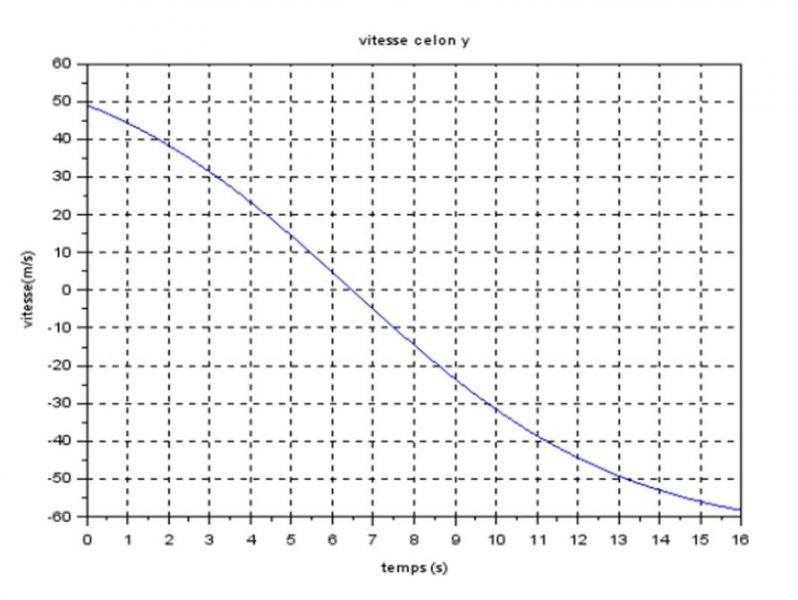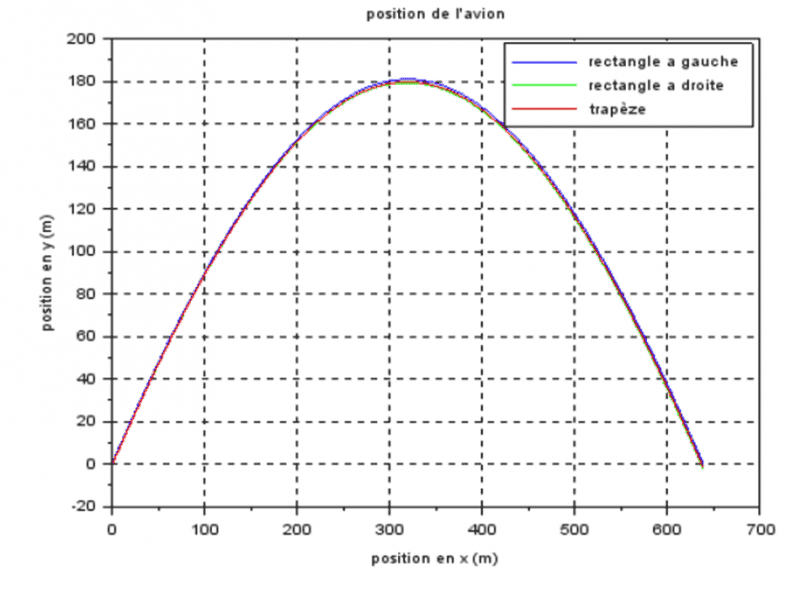# Acceleration to position

• Buzz23

#### Buzz23

Hello
I've just subscribe because I've a problem with a project.
First, I'm a student in France, in prep school. My team and I are working on a project : we study the perfect path for an airplane to be in micro-gravity (it means the only acceleration is the one due to gravity, like what we have here :http://www.airzerog.com/)

So, in order to verify our theoretical model, we experienced in an aerobatic plane (CAP10) and we had a accelerometer installed in the plane (desc of the accelerometer :http://www.gcdataconcepts.com/GCDC_X16-1D_User_Manual.pdf). It's a 3 axis accelerometer, which returns the measures in a CSV file with : time-accX-accY-accZ)

The purpose of those measures were to obtain the path followed by the plane, to actually see the parabola. I did a program on MATLAB :
-to import the measures from the csv file and pu them into variables (it works fine)
-to filter it (with a butterworth filter), because the signal was very noisy, due to the plane's vibration) (it works fine too)
-and then to integer it, twice. And here is the problem : the program run well, but it returns ironical values ; when we begun our parabola at 610 m (2000ft), it ends in the abyssal (approximatly -2000m..).

I don't understand why. I used the cumptrapz function of MATLAB (i just wanted an idea of the path, not a very precise one). I also converted all the mesaures, after having filtered them, to SI system. I multiplied them to 1/2048(accelerometer's gain), then to 9.81 to have it in m/s². I didn't forgot the constant when i integrated (610 m and v0 m/s).
As we did several parabola (10) with different initial velocity (remember : the purpose was to see the influence of several factors such as the velocity and the angle with which we began the parabola), i ran the algorithm with different parabola and there's always the same mistake.

Please find in attachment my different programs:
-importation(i) import the measures into different variable
-filtrage_butterworth filter my measures
-integrale_trapz integrate the signal
-trajectoire return the position to Z and X in order to plot(X,Z), and temps (the vector time) to plot(temps,Z)

Thank you for reading me and I would appreciate any kind off commentaries to my code.
Buzz23

PS : please forgive my approximate english :)

#### Attachments

• Trajectoire.txt
667 bytes · Views: 475
• filtrage_butterworth.txt
212 bytes · Views: 499
• importation.txt
370 bytes · Views: 476
• integrale_trapz.txt
412 bytes · Views: 484
Do you have an example path with accelerations, velocities and positions? The code has many magic numbers and conversions that are hard to understand, data would help to see where the error comes from.

The gravity vector should be -9.81 pointing down. I can't tell from the code whether your gravity vector is pointing up or down... the code shows +9.81?

Here are some samples we had from our theoretical analysis.
-velocity on z axis (up)
-path : x=f(z)
-z acceleration :http://www.google.fr/imgres?imgurl=...r=3072&page=1&start=0&ndsp=37&ved=0CC4QrQMwBA (the blue one)The gravity vector should be -9.81 pointing down. I can't tell from the code whether your gravity vector is pointing up or down... the code shows +9.81?

No, during the period of micro-gravity, the value for the acceleration on z is 0.

No, during the period of micro-gravity, the value for the acceleration on z is 0.
But the gravity component doesn't change! When you are flying a curve, there is an additional centripetal acceleration component, to bring the resultant to 'zero'.

No, during the period of micro-gravity, the value for the acceleration on z is 0.
That's what your accelerometer will read. The real airplane will still accelerate downwards.
The velocity/time-diagram does not show a microgravity environment.

That's what your accelerometer will read. The real airplane will still accelerate downwards.
The velocity/time-diagram does not show a microgravity environment.
So what do I have to do? Add to my measure on Z the gravity?

Sure.

Also, how did you convert the three acceleration measurements to your reference frame? The plane will change its position, if you don't have some special mechanism the accelerometer axes won't be aligned with the coordinates all the time.

That's what I thougt! I'm actually trying to place them in the correct reference(Earth), whether they are measured in the reference of the plane.

So I also have to substract the Earth attraction.. I put the Z axis down, do I have to add 9.81, or substract 9.81?

Consider the case where the accelerometers read zero, but the airplane is accelerating downwards...